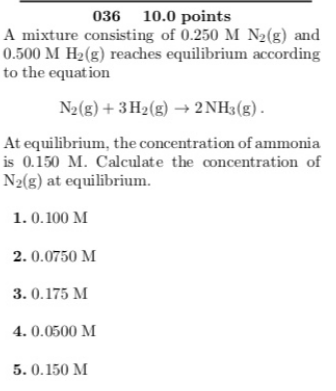# A mixture consisting of 0.250 M N2(g) and 0.500 M H2(g) reaches equilibrium according to the equation N2(g) + 3 H2(g) → 2NH3(g). At equilibrium, the concentration of ammonia is 0.150 M. Calculate the concentration of N2(g) at equilibrium. 1. 0.100 M 2. 0.0750 3. 0.175 M 4. 0.0500 M 5. 0.150 M